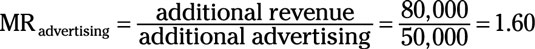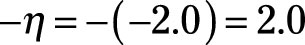##### Managerial Economics For Dummies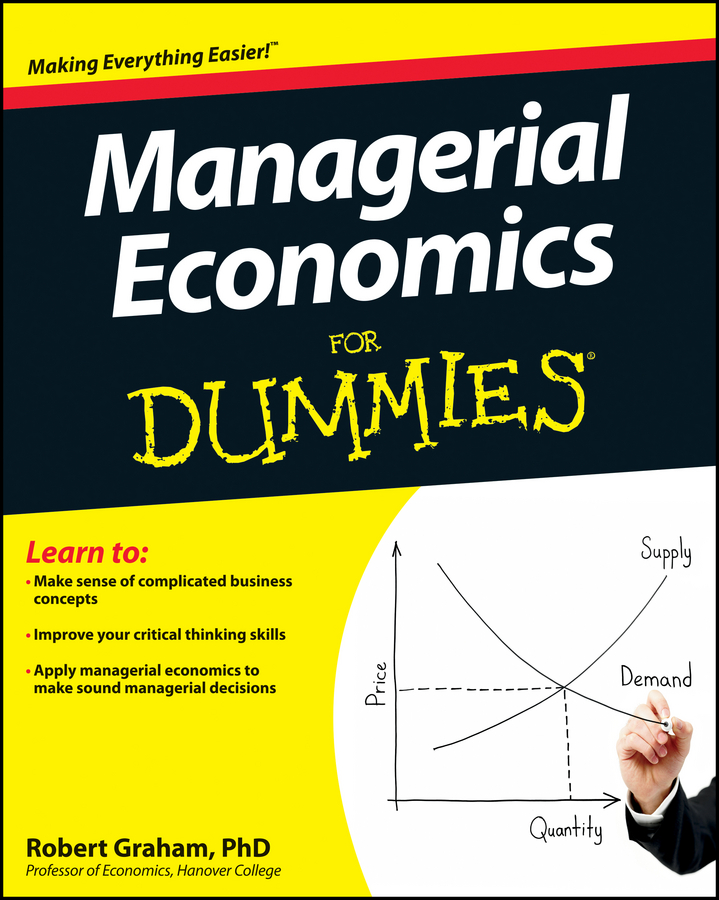Managerial economic theory indicates that one factor contributing to product differentiation in monopolistically competitive markets is advertising. Determining the appropriate or profit-maximizing level of advertising is crucial to the firm’s success.

Advertising’s affect on consumer taste and preferences enables it to influence consumer demand for a product. For the monopolistically competitive firm, an increase in advertising increases demand for its product, while a decrease in advertising decreases the firm’s demand. However, in addition to shifting demand, you must recognize that changes in advertising expenditures affect your cost.

Advertising increases your profit as long as the marginal revenue obtained through the sale of additional units is greater than the marginal cost of producing those units plus the cost of the additional advertising expenditures.

Mathematically, the additional profit that’s made through the production and sale of one additional unit of output is price minus marginal cost, PMC. The change in gross profit that occurs with an additional dollar spent on advertising equals the change in the quantity sold that occurs, Δq, multiplied by PMC. This amount is gross profit, because it doesn’t include the cost of advertising.

The monopolistically competitive firm’s net profit increases as long as each additional dollar spent on advertising generates more than an additional dollar’s worth of gross profit. In other words, net profit increases as long as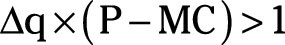In order to maximize the net profits associated with advertising, the firm should continue increasing advertising expenditures until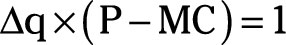At this point, an additional dollar’s worth of advertising adds one dollar to gross profit, resulting in no gain in the firm’s net profit.

Here the previous equation is manipulated to derive a very important relationship. If you want to skip the manipulation, that’s fine. Go straight to the result, and trust that the relationship really works.

If you take the previous equation and divide both sides by (PMC), you get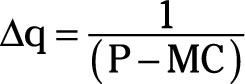Now, multiplying both sides of the equation by P yields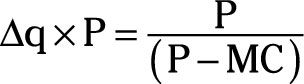At the point of profit maximization, marginal revenue equals marginal cost. Substituting MR for MC in the equation leads to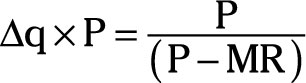The right side of this equation equals the negative price elasticity of demand, ç, for the firm’s product. The left side of the equation represents the marginal revenue of an additional dollar’s worth of advertising. Therefore, the optimal level of advertising expenditures corresponds to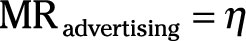Wow, there’s the price elasticity of demand again. Maybe mothers should stop saying, “Just worry about yourself,” and instead say, “Just remember your price elasticity of demand.”

You believe the price elasticity of demand for your product is –2.0. Your advertising department is requesting an additional \$50,000 of advertising, arguing that the additional advertising will increase sales by \$80,000. In order to determine whether or not to support the advertising department’s request, you take the following steps:

1. Determine the marginal revenue of an additional dollar’s worth of advertising.